# How to calculate age from date of birth

This post explains that how to get age from the date of birth in a Cell. How to calculate birthdate to age using formulas in Excel. How to convert the birth of date to age quickly in Excel.

## Calculate age from date of birth

If you have a table that contains birthdate data in a range of Cells in Excel, and you want to get the age from those people’s birthdate value, you can use a combination of the INT function, the TODAY function to create an excel formula .

You can use the TODAY function to get the current date and then is subtracted to the value of birthdate and then the number is divided by 365 to get the exact age value, last, using the INT function to convert the age value to the integer.

Assuming that you want to calculate date of birth to age in Cell B2, you can use the following formula:

`=INT((TODAY()-B2)/365)`

Let’s see how this formula works:

=TODAY()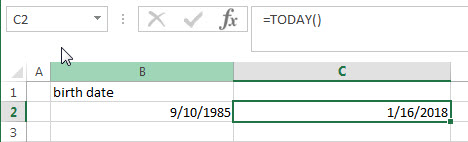This formula returns the current date.

=(TODAY()-B2)/365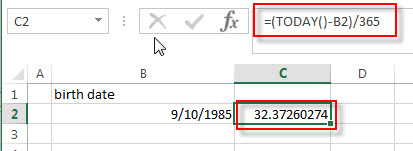The current date subtracts the date of birth to get the number of days of birth.  The returned number is divided by 365 (one year) to get the exact numbers of years.  Then it goes into the INT function as its argument.

=INT((TODAY()-B2)/365)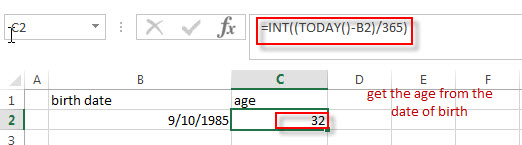This formula will round a decimal down to an integer. So you can get the age from the date of birth.

## Calculate age from date of birth with DATEDIF function

You can also create a formula based on the DATEIF function and TODAY function or NOW function. The DATEDIF function can return the number of complete year between the current date and birth date. So you can write down the following formula to convert date of birth to age quickly:

`=DATEDIF(B2,TODAY(),"Y")`

OR

`=DATEDIF(B2,NOW(),"Y")`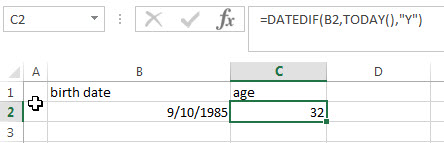## Calculate age from date of birth with YEARFRAC function

You can also create an excel formula based on the INT function or ROUNDDOWN function, the YEARFRAC function and the TODAY function to calculate age from birth of date.

The YEARFRAC function can be used to calculate the fraction of the year represented by the number of whole days between the date of birth and the current date.  Then you need to use the INT function or ROUNDDOWN function to round down the number returned by the YEARFRAC function to the integer value.

So you can write down the following formula:

`=INT(YEARFRAC(B2, TODAY(), 1))`

OR

`=ROUNDDOWN(YEARFRAC(B2, TODAY(), 1),0)`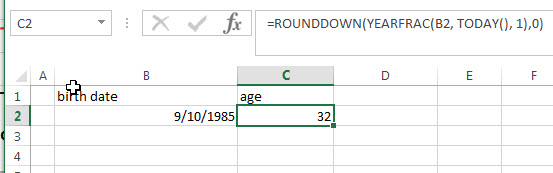### Related Formulas

• Convert Date to Day of Week in Excel
If you want to get the day name of the week as an abbreviation from a date, such as: Mon, Tue, etc. Enter the following TEXT formula in the Cell C1 that you want to get the day name of the week…
• Convet Text Date dd/mm/yy to mm/dd/yyyy as Date Format
If you want to convert dates in the text format to the date format as mm/dd/yyyy, you can use a combination of the DATE function, the VALUE function, the RIGHT function, the MID function, and the LEFT function to create a new excel formula….
• Convert date to month name with Text Function
If you want to convert the date to a month name, you can use the TEXT function with a specified format code like “mmm”in excel.  You can try to run the following TEXT formula:=TEXT(B1,”mmm”)…
• Convert Date to text with Text Function in Excel
you can use TEXT function in excel to convert dates to text in a specific format code. For example, The TEXT function can use the following patterns, like as: “mm/dd/yyyy”, “yyyy/mm/dd”, etc…
• Convert date to month and year only in excel
If you want to convert the date to month and year only, you can use “yyyymm” format code within the TEXT function in excel, so you can write down the below TEXT formula:=TEXT(date,”yyyymm”)…,
• Convert date to month and day only in excel
If you want to convert the date (mm/dd/yyyy) to month and day only, you can use “mm dd” format code within the TEXT function in excel, so you can write down the below TEXT formula: =TEXT(date,”mm dd”)…

### Related Functions

• Excel DATEDIF function
The Excel DATEDIF function returns the number of days, months, or years between tow dates.The syntax of the DATEDIF function is as below:=DATEDIF (start_date,end_date,unit)…
• Excel INT function
The Excel INT function returns the integer portion of a given number. And it will rounds a given number down to the nearest integer.The syntax of the INT function is as below:= INT (number)…